常用集合类相关知识点总结

2021-08-17 13:36:2343

前言

HashMap的是怎么扩容的，它的Hash值是怎么计算的？index位置又是如何计算的？为什么要这么算？为什么扩容是 2 倍 而不是 3 倍、4 倍呢？重写的hashCode 有什么作用？

目录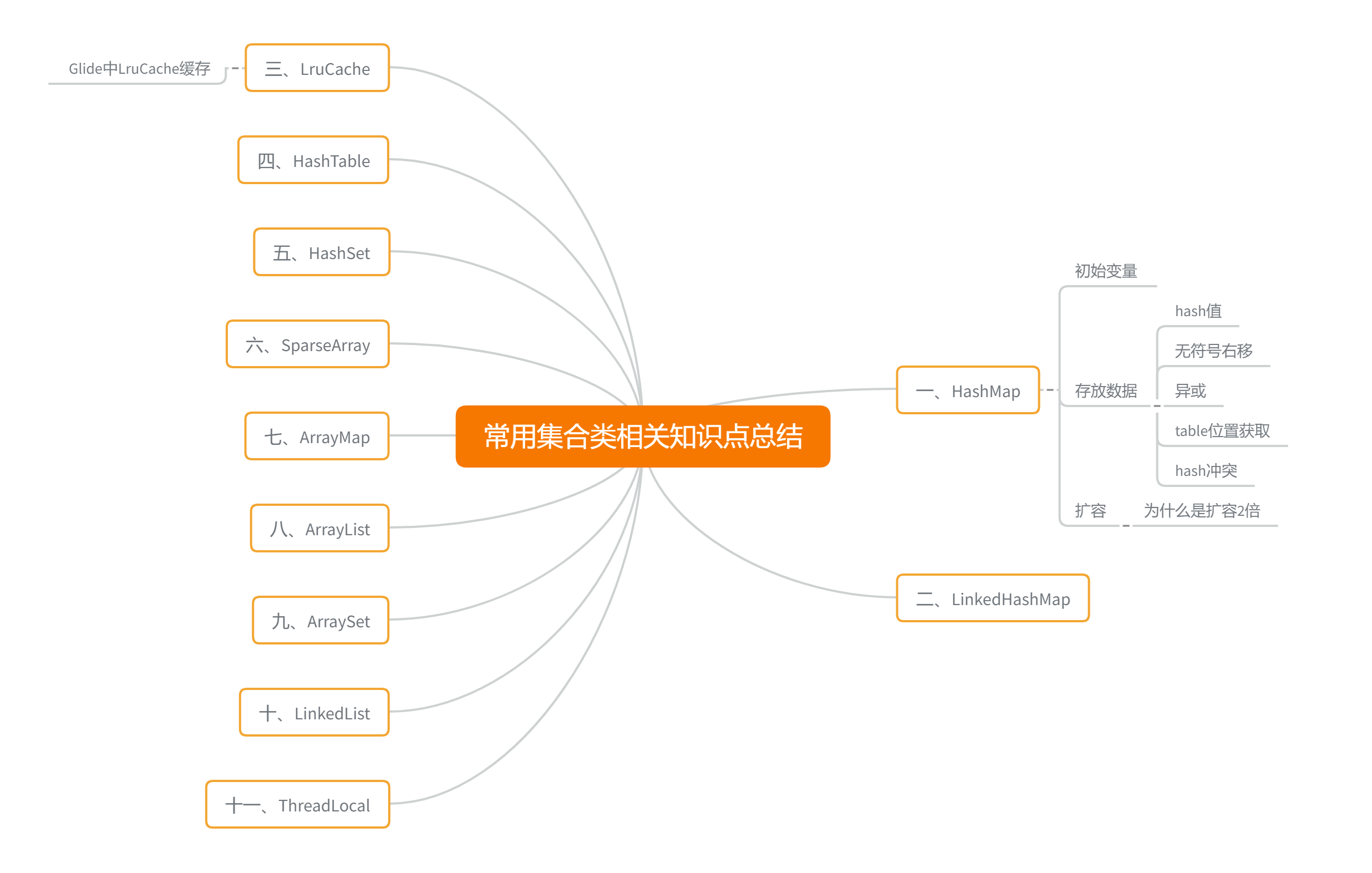一、HashMap

1、初始变量

threshold = 16 * 0.75 = 12 在存储完元素后会根据该值判断是否需要扩容

table size = 16

TREEIFY_THRESHOLD = 8 链表转换成红黑树时机

UNTREEIFY_THRESHOLD = 6 红黑树转换成链表时机

MIN_TREEIFY_CAPACITY = 64 树行化时机

public HashMap() {
}
resize() 方法

newCap = DEFAULT_INITIAL_CAPACITY;
Node<K,V>[] newTab = (Node<K,V>[])new Node[newCap];

2、存放数据

put(K key, V value)

public V put(K key, V value) {
return putVal(hash(key), key, value, false, true);
}

static final int hash(Object key) {
int h;
return (key == null) ? 0 : (h = key.hashCode()) ^ (h >>> 16);
}

final V putVal(int hash, K key, V value, boolean onlyIfAbsent,
boolean evict) {
Node<K,V>[] tab; Node<K,V> p; int n, i;
if ((tab = table) == null || (n = tab.length) == 0)
n = (tab = resize()).length;
if ((p = tab[i = (n - 1) & hash]) == null)
// 注释 1 如果 对应存储位置没有存Node数据
tab[i] = newNode(hash, key, value, null);
else {
Node<K,V> e; K k;
if (p.hash == hash &&
((k = p.key) == key || (key != null && key.equals(k))))
e = p; //注释 2 如果 找到了对应的key则更新数据
else if (p instanceof TreeNode)//注释 2 如果 是树节点 进行红黑数操作，后面分析
e = ((TreeNode<K,V>)p).putTreeVal(this, tab, hash, key, value);
else {
for (int binCount = 0; ; ++binCount) {
if ((e = p.next) == null) {
//注释 3  寻找最后一个节点 给next节点创建Node
p.next = newNode(hash, key, value, null);
//注释 4 如果 bitCount >= 8 - 1 ,转换成红黑树
if (binCount >= TREEIFY_THRESHOLD - 1) // -1 for 1st
treeifyBin(tab, hash);
break;
}
if (e.hash == hash &&
((k = e.key) == key || (key != null && key.equals(k))))
break;
p = e;//交换 下一轮for 操作
}
}
if (e != null) { // existing mapping for key
V oldValue = e.value;
if (!onlyIfAbsent || oldValue == null)
e.value = value;
afterNodeAccess(e);
return oldValue;
}
}
++modCount;
if (++size > threshold)
resize();
afterNodeInsertion(evict);
return null;
}

n是 2 的幂次方时，两者结果一样，& 操作 比 取余 运算速度快，因为取余操作，底层也要转换成位运算。因为key.hashCode取出来的hash值不是数组的索引下标，为了能随机的计算出索引下标，常见的hash算法就是取模。

hash冲突怎么处理？

3、扩容

java 8 之前，HashMap初始化时会默认容量16，java 8 中，HashMap默认容量为 0，只有第一次put元素时，才会扩容到 16。

if ((newCap = oldCap << 1) < MAXIMUM_CAPACITY && oldCap >= DEFAULT_INITIAL_CAPACITY)
newThr = oldThr << 1;

for (int j = 0; j < oldCap; ++j) {
Node<K,V> e;
if ((e = oldTab[j]) != null) {
oldTab[j] = null;
if (e.next == null)
newTab[e.hash & (newCap - 1)] = e;
else if (e instanceof TreeNode)
((TreeNode<K,V>)e).split(this, newTab, j, oldCap);
else { // preserve order
Node<K,V> loHead = null, loTail = null;
Node<K,V> hiHead = null, hiTail = null;
Node<K,V> next;
do {
next = e.next;
if ((e.hash & oldCap) == 0) {
if (loTail == null)
else
loTail.next = e;
loTail = e;
}
else {
if (hiTail == null)
else
hiTail.next = e;
hiTail = e;
}
} while ((e = next) != null);
if (loTail != null) {
loTail.next = null;
}
if (hiTail != null) {
hiTail.next = null;
}
}
}
}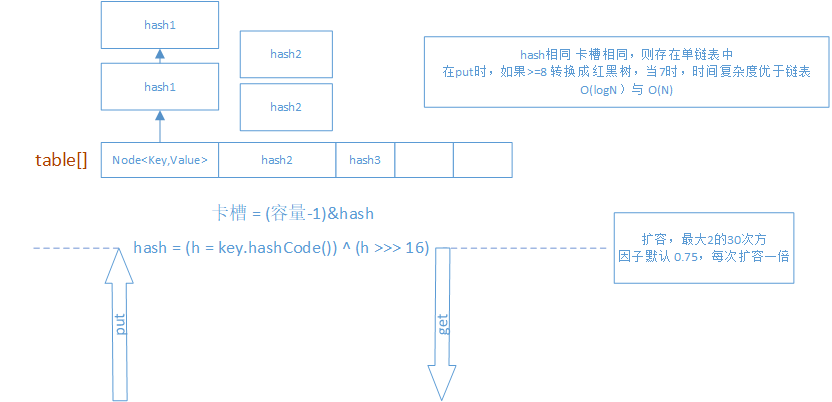put元素时，先会放到链表Node节点的next指针上，然后更换节点的afterbefore指向，将该元素放到链表最后。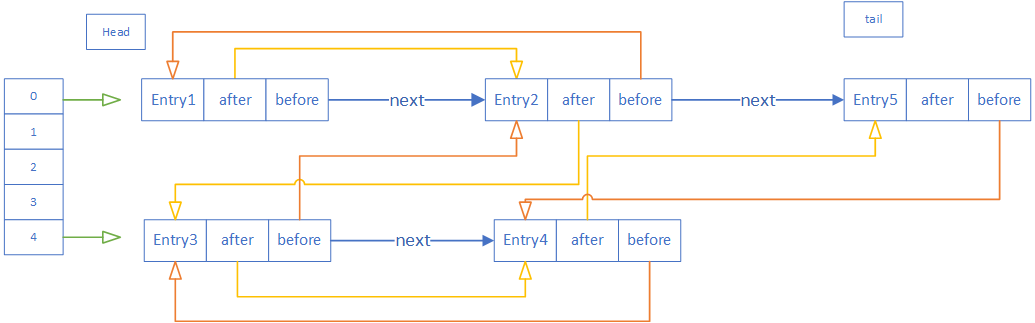三、LruCache

public class MyLruCache<K, V> {
private int cacheSize;
private float factory = 0.75f;
​
public MyLruCache(int cacheSize) {
this.cacheSize = cacheSize;
cacheMap = new LinkedHashMap<>(cacheSize, factory, true);
}
​
public V get(K key) {
if (cacheMap.containsKey(key)) {
return cacheMap.get(key);
}
return null;
}
​
public void put(K key, V value) {
cacheMap.put(key, value);
}

}

LruCache就是一个持有一定数量强引用数据的缓存。当访问一个数据时，这个数据就会被移动到数据队列的头部(经常用到的数据)，当数据添加到缓存满时，队列尾部的数据(也就是不常用到的数据)会被删除并被回收。

synchronized (this) {
mapValue = map.get(key);
if (mapValue != null) {
hitCount++;
return mapValue;
}
missCount++;
}

put方法中，每次都会执行 trimToSize 方法，利用while死循环，来找到链表末尾的数据，并删除。查找代码如下

Map.Entry<K, V> toEvict = null;
for (Map.Entry<K, V> entry : map.entrySet()) {//LinkedHashMap是有序的
toEvict = entry;
}
// END LAYOUTLIB CHANGE
​
if (toEvict == null) {
break;
}
​
key = toEvict.getKey();
value = toEvict.getValue();
map.remove(key);

Glide中LruCache缓存是如何做的？(后续会专门分析Glide的编解码和缓存)

public synchronized Y put(@NonNull T key, @Nullable Y item) {
final int itemSize = getSize(item);
if (itemSize >= maxSize) {  //注释1 所有存入的Bitmap的大小要比你设定的最大缓冲值还大
onItemEvicted(key, item);   // 这个方法是提供给子类用，当前Bitmap被清除
return null;
}
​
if (item != null) {
currentSize += itemSize; // 注释2 记录当前大小
}
@Nullable final Y old = cache.put(key, item); //注释 3 这里就是LinkedHashMap put了，把元素放到链表队列末尾
if (old != null) {
currentSize -= getSize(old);
​
if (!old.equals(item)) {
onItemEvicted(key, old); //注释3 清楚old bitmap
}
}
evict(); //注释4 这里调用的是 trimToSize 开始限制容量
​
return old;
}

protected synchronized void trimToSize(long size) {
Map.Entry<T, Y> last;
Iterator<Map.Entry<T, Y>> cacheIterator;
while (currentSize > size) {
cacheIterator  = cache.entrySet().iterator();
last = cacheIterator.next();
final Y toRemove = last.getValue();
currentSize -= getSize(toRemove);
final T key = last.getKey();
cacheIterator.remove();
onItemEvicted(key, toRemove);
}
}

四、HashTable

HashTable每次找到数据，如果没有在table相应index中存储，将会把数据直接放到链表头

HashtableEntry<K,V> e = (HashtableEntry<K,V>) tab[index];
tab[index] = new HashtableEntry<>(hash, key, value, e);

六、SparseArray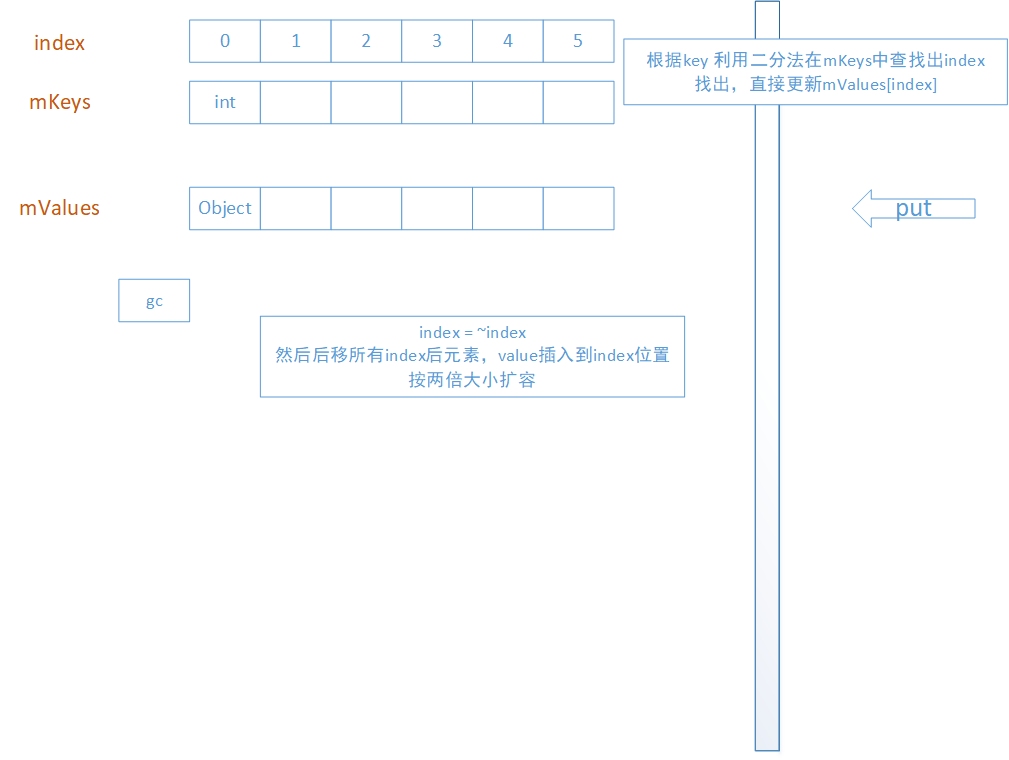**1、**如果调用remove删除元素后，元素会标记DELETE状态，在下一次put元素时，如果容量超过了mKeys.length，会执行gc方法，将mKeysmValues中那些标记了DELETE状态的元素元素删除，数据整体前移。

private void gc() {
int n = mSize;
int o = 0;
int[] keys = mKeys;
Object[] values = mValues;
​
for (int i = 0; i < n; i++) {
Object val = values[i];
​
if (val != DELETED) {
if (i != o) {
keys[o] = keys[i];
values[o] = val;
values[i] = null;
}
​
o++;
}
}
mGarbage = false;
mSize = o;
}

**2、**如果put元素时，没有找到对应的下标，将会对计算出的索引值index取反，返回值要么是0，要么为负数，但是插入数据前也会进行取反操作。通过二分法这种方式，mKeys内部的值一直是按照递增的方式来排列的，因为下面代码的 lo 它最后结果是 lo = hi + 1，所以插入元素到 lo 位置时，后面元素需要整体后移。

static int binarySearch(int[] array, int size, int value) {
int lo = 0;
int hi = size - 1;
​
while (lo <= hi) {
final int mid = (lo + hi) >>> 1;
final int midVal = array[mid];
​
if (midVal < value) {
lo = mid + 1;
} else if (midVal > value) {
hi = mid - 1;
} else {
return mid;  // value found
}
}
return ~lo;  // value not present
}

**3、**没有找到下标，是如何存放元素，即扩容是怎么扩的

public static <T> T[] insert(T[] array, int currentSize, int index, T element) {
if (currentSize + 1 <= array.length) {
System.arraycopy(array, index, array, index + 1, currentSize - index);
array[index] = element;
return array;
}
​
T[] newArray = (T[]) Array.newInstance(array.getClass().getComponentType(),
growSize(currentSize));
System.arraycopy(array, 0, newArray, 0, index);
newArray[index] = element;
System.arraycopy(array, index, newArray, index + 1, array.length - index);
return newArray;
}

七、ArrayMap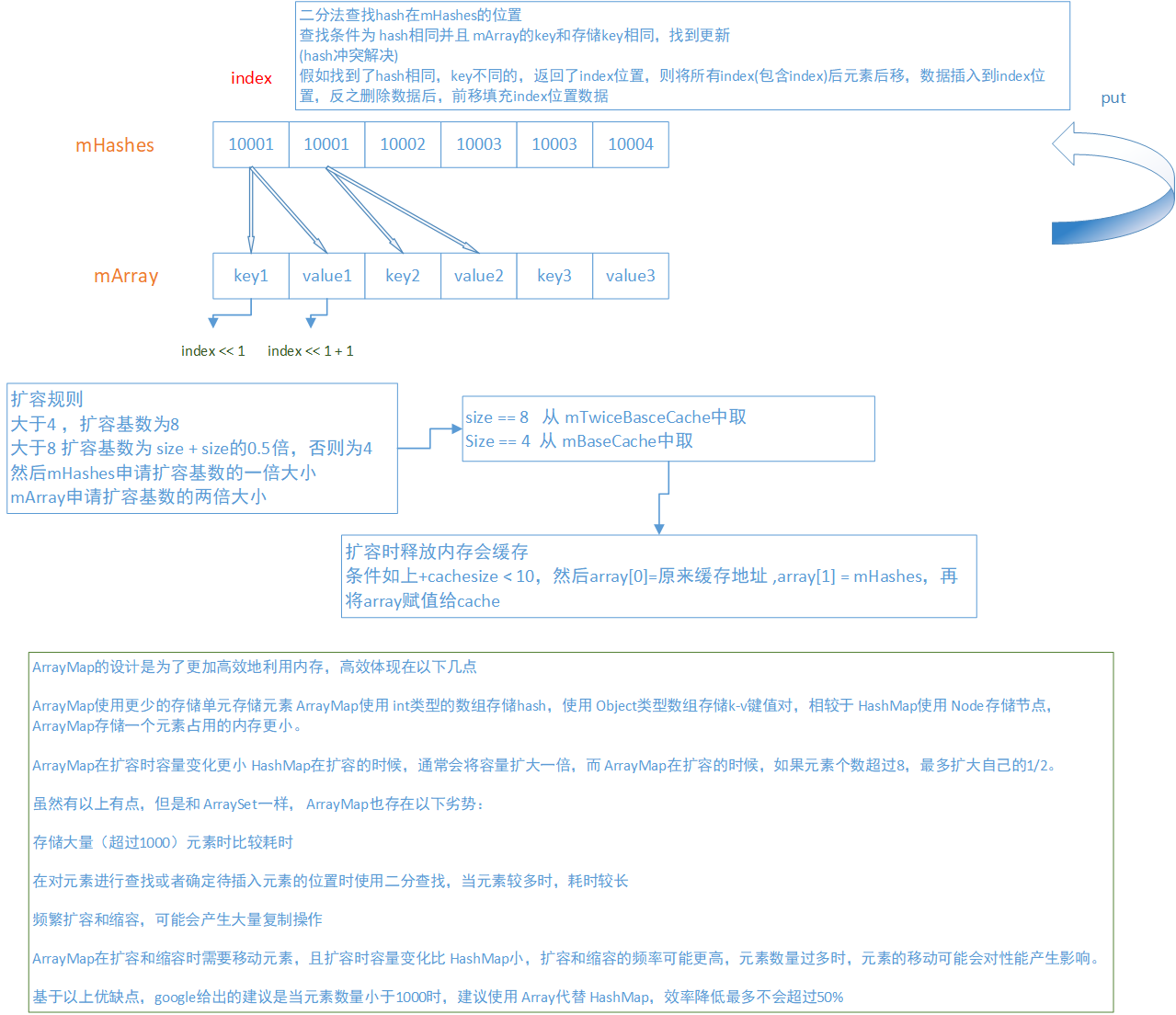keynull时，hash = 0，也就是说key = nullmArray存放对应的值。通过new ArrayMap() 方式使用，hash值就是 key.hashCode() ,然后得到该hashmHash中的位置。这个有点类似SparseArray了。

put元素时，如果大小已经超过了mHashes.length，将会判断扩容大小

final int n = osize >= (BASE_SIZE*2) ? (osize+(osize>>1))
: (osize >= BASE_SIZE ? (BASE_SIZE*2) : BASE_SIZE); //BASE_SIZE = 4

private static void freeArrays(final int[] hashes, final Object[] array, final int size) {
if (hashes.length == (BASE_SIZE*2)) {
synchronized (ArrayMap.class) {
if (mTwiceBaseCacheSize < CACHE_SIZE) {
// 将传过来的 array和array分别保存 mTwiceBaseCache和 hashes
array = mTwiceBaseCache;
array = hashes;
//array所有数据 除了 下标 0 1 的数据都置空
for (int i=(size<<1)-1; i>=2; i--) {
array[i] = null;
}
//mTwiceBaseCache 指向 array
mTwiceBaseCache = array;
mTwiceBaseCacheSize++;
if (DEBUG) Log.d(TAG, "Storing 2x cache " + array
+ " now have " + mTwiceBaseCacheSize + " entries");
}
}
} else if (hashes.length == BASE_SIZE) {
// mBaseCache
}

mTwiceBaseCache结构如下图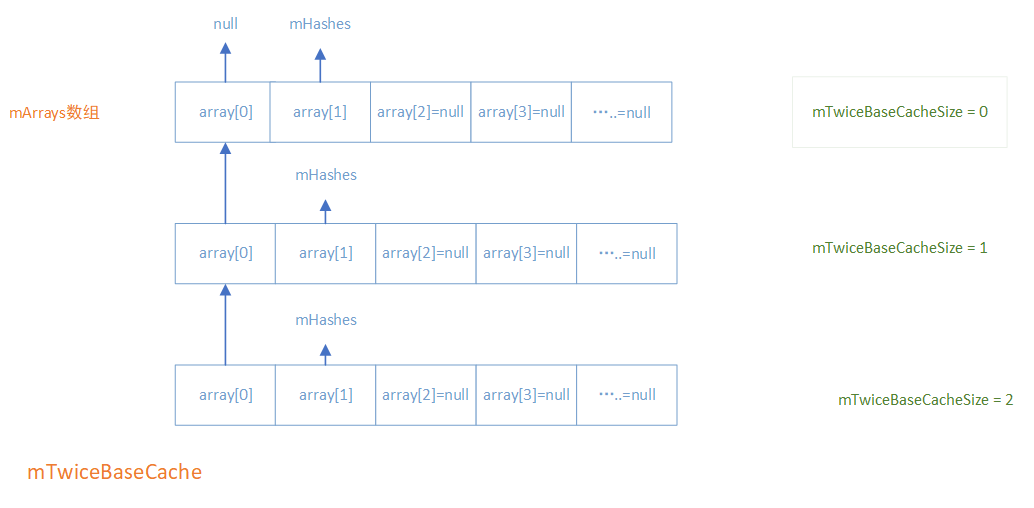private void allocArrays(final int size) {
if (mHashes == EMPTY_IMMUTABLE_INTS) {
throw new UnsupportedOperationException("ArrayMap is immutable");
}
if (size == (BASE_SIZE*2)) {
synchronized (ArrayMap.class) {
if (mTwiceBaseCache != null) {
//这里其实就是设置缓存的逆过程
final Object[] array = mTwiceBaseCache;
mArray = array;
mTwiceBaseCache = (Object[])array;
mHashes = (int[])array;
array = array = null;
mTwiceBaseCacheSize--;
if (DEBUG) Log.d(TAG, "Retrieving 2x cache " + mHashes
+ " now have " + mTwiceBaseCacheSize + " entries");
return;
}
}
} else if (size == BASE_SIZE) {
//mBaseCache
}
​
mHashes = new int[size];
mArray = new Object[size<<1];
}

九、ArraySet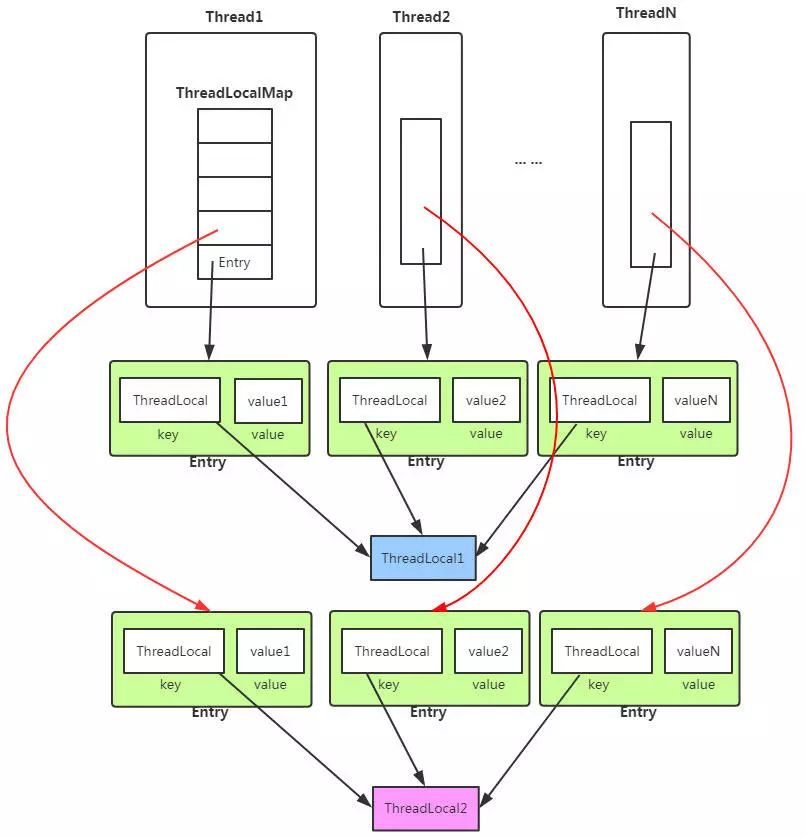public void set(T value) {
if (map != null)
map.set(this, value);
else
createMap(t, value);
}

private void set(ThreadLocal<?> key, Object value) {

// We don't use a fast path as with get() because it is at
// least as common to use set() to create new entries as
// it is to replace existing ones, in which case, a fast
// path would fail more often than not.

Entry[] tab = table;
int len = tab.length;
int i = key.threadLocalHashCode & (len-1);

for (Entry e = tab[i];
e != null;
e = tab[i = nextIndex(i, len)]) {

if (k == key) {
e.value = value;
return;
}

if (k == null) {
replaceStaleEntry(key, value, i);
return;
}
}

tab[i] = new Entry(key, value);
int sz = ++size;
if (!cleanSomeSlots(i, sz) && sz >= threshold)
rehash();
}苏公网安备 32030302000848号   苏ICP备20033101号-1